Complex Numbers & Quadratic Equations- 1

# Complex Numbers & Quadratic Equations- 1 - Commerce

Test Description

## 25 Questions MCQ Test Mathematics (Maths) Class 11 - Complex Numbers & Quadratic Equations- 1

Complex Numbers & Quadratic Equations- 1 for Commerce 2023 is part of Mathematics (Maths) Class 11 preparation. The Complex Numbers & Quadratic Equations- 1 questions and answers have been prepared according to the Commerce exam syllabus.The Complex Numbers & Quadratic Equations- 1 MCQs are made for Commerce 2023 Exam. Find important definitions, questions, notes, meanings, examples, exercises, MCQs and online tests for Complex Numbers & Quadratic Equations- 1 below.
Solutions of Complex Numbers & Quadratic Equations- 1 questions in English are available as part of our Mathematics (Maths) Class 11 for Commerce & Complex Numbers & Quadratic Equations- 1 solutions in Hindi for Mathematics (Maths) Class 11 course. Download more important topics, notes, lectures and mock test series for Commerce Exam by signing up for free. Attempt Complex Numbers & Quadratic Equations- 1 | 25 questions in 25 minutes | Mock test for Commerce preparation | Free important questions MCQ to study Mathematics (Maths) Class 11 for Commerce Exam | Download free PDF with solutions
 1 Crore+ students have signed up on EduRev. Have you?
Complex Numbers & Quadratic Equations- 1 - Question 1

### The complex numbers z = x + iy which satisfy the equation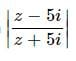= 1 lie on

Detailed Solution for Complex Numbers & Quadratic Equations- 1 - Question 1

∣z−5i∣/∣z+5i| = 1
∣z−5i∣=∣z+5i| (Using definition ∣z−z1∣=∣z−z2∣ given Perpendicular bisector of z1 and z2.)
⇒ Perpendicular bisector of points (0,5) and (0,−5). which lies on x-axis.

Complex Numbers & Quadratic Equations- 1 - Question 2

### The inequality | z − 4 | < | z −2 | represents the region given by

Detailed Solution for Complex Numbers & Quadratic Equations- 1 - Question 2

Let, z = x + iy
Putting in the given inequality,
|(x−4)−iy| < |(x−2)+iy|
⇒ [(x−4)2 + y2]11/2 < [(x−2)2 + y2]1/2
squaring both sides,
⇒ (x−4)2 + y2 < (x−2)2 + y2
⇒ −8x+16 < −4x+4
⇒ 4

Complex Numbers & Quadratic Equations- 1 - Question 3

###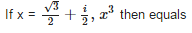Detailed Solution for Complex Numbers & Quadratic Equations- 1 - Question 3

x = (√3+i)/2
x3 = 1/8(√3+i)3
Apply formula (a+b)3 = a3 + b3 + 3a2b + 3ab2
= (3√3 + i3 + 3*3*i + 3*√3*i2)/8
= (3√3 - i + 9i - 3√3)/8
= 8i/8
= i

Complex Numbers & Quadratic Equations- 1 - Question 4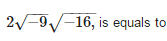Complex Numbers & Quadratic Equations- 1 - Question 5

If (√3 + i)10 = a + ib: a, b ∈ R, then a and b are respectively :

Detailed Solution for Complex Numbers & Quadratic Equations- 1 - Question 5

(√3 + i)10 = a + ib
Z = √3 + i = rcosθ + i rsinθ
⇒ √3 = rcosθ   i = rsinθ
⇒ (√3)2 + (1)2 = r2cos2θ + r2sin2θ
⇒ 4 = r2
⇒ r = 2
tan = 1/√3
⇒ tan π/6
Therefore, Z = √3 + i = 2(cos π/6 + i sin π/6)
(Z)10 = √3 + i = (2cos π/6 + 2i sin π/6)10
= 210 (cos π/6 + i sin π/6)10
210 (cos 10π/6 + i sin 10π/6)
= 210 (cos(2π - π/3) + i sin(2π - π/3))
= 210 (cos π/3 - i sin π/3)
= 210 (1/2 - i√3/2)
29(1 - i√3)
a = 29 = 512
b = - 29(√3) = -512√3

Complex Numbers & Quadratic Equations- 1 - Question 6

Let x,y ∈ R, hen x + iy is a non real complex number if

Complex Numbers & Quadratic Equations- 1 - Question 7

Let x,y ∈ R, then x + iy is a purely imaginary number if

Complex Numbers & Quadratic Equations- 1 - Question 8

Multiplicative inverse of the non zero complex number x + iy (x,y ∈ R,)

Detailed Solution for Complex Numbers & Quadratic Equations- 1 - Question 8

Let u be multiplicative inverse
zu = 1
u = 1/z
u = 1/(x+iy)
Rationalise it
[1/(x+iy)]*[(x-iy)/(x-iy)]
= (x-iy)/(x2+y2)
u = x/(x2+y2) +i(-y)/(x2+y2)

Complex Numbers & Quadratic Equations- 1 - Question 9

The locus of z which satisfied the inequality log0.5|z –2| > log0.5|z – i| is given by

Complex Numbers & Quadratic Equations- 1 - Question 10

The inequality | z − 6 | < | z − 2 | represents the region given by

Detailed Solution for Complex Numbers & Quadratic Equations- 1 - Question 10

Let, z = x + iy

Putting in the given inequality,

∣(x−4) − iy∣ < ∣(x−2) + iy∣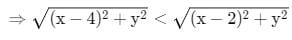squaring both sides,

⇒ (x−4)+ y< (x−2)+ y2
⇒ −8x + 16 < −4x + 4
⇒ 4x > 12
⇒ x > 3
⇒ Re(z) > 3

Complex Numbers & Quadratic Equations- 1 - Question 11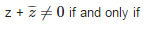Complex Numbers & Quadratic Equations- 1 - Question 12

Distance of the representative of the number 1 + I from the origin (in Argand’s diagram) is

Complex Numbers & Quadratic Equations- 1 - Question 13

If ω is a cube root of unity , then (1+ω)(1+ω2)(1+ω4)(1+ω8)...... upto 2n factors is

Complex Numbers & Quadratic Equations- 1 - Question 14

If points corresponding to the complex numbers z1, z2, z3 and z4 are the vertices of a rhombus, taken in order, then for a non-zero real number k

Detailed Solution for Complex Numbers & Quadratic Equations- 1 - Question 14

AC = z3 = z1 eiπ
= z1 (cosπ + i sinπ)
= z3 = z1(-1 + i(0))
= z3 = -z1
AC = z1 - z3
BC = z2 - z4
(z1 - z3)/(z2 - z4) = k
(z1 - z3) = eiπ/2(z2 - z4)
(z1 - z3) k(cosπ/2 + sinπ/2) (z2 - z4)
z1 - z3 = ki(z2 - z4)
z1 - z3 = ik(z2 - z4)

Complex Numbers & Quadratic Equations- 1 - Question 15

If k , l, m , n are four consecutive integers, then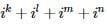is equal to :

Complex Numbers & Quadratic Equations- 1 - Question 16

i2+i4+i6+........... up to 2k + 1 terms, for all k belongs to natural numbers N.

Complex Numbers & Quadratic Equations- 1 - Question 17

1+i+i2+i3+...... up to 4n terms is equal to

Complex Numbers & Quadratic Equations- 1 - Question 18

If |z1| = 4, |z2| = 4, then |z1 + z2 + 3 + 4i| is less than

Complex Numbers & Quadratic Equations- 1 - Question 19

If If ω is a non real cube root of unity and (1+ω)= a+bω;a,b ∈ R, then a and b are respectively the numbers :

Detailed Solution for Complex Numbers & Quadratic Equations- 1 - Question 19

Since w is the cube root of unity.
(1+w)9 =A+Bw
⇒(−w2)9 =A+Bw
⇒−(w3)6 = A+Bw
⇒−1=A+Bw
∴ A= -1 & B=0

Complex Numbers & Quadratic Equations- 1 - Question 20

If | z | = 1, then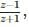z ≠ -1 is

Complex Numbers & Quadratic Equations- 1 - Question 21

In z = a + bι, if i is replaced by −ι, then another complex number obtained is said to b

Complex Numbers & Quadratic Equations- 1 - Question 22

If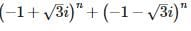= 2n α, then α is equal to :

Complex Numbers & Quadratic Equations- 1 - Question 23

If (x + iy) (3 - 4i) = (5 + 12i), then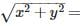Complex Numbers & Quadratic Equations- 1 - Question 24

The locus of the equation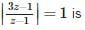Detailed Solution for Complex Numbers & Quadratic Equations- 1 - Question 24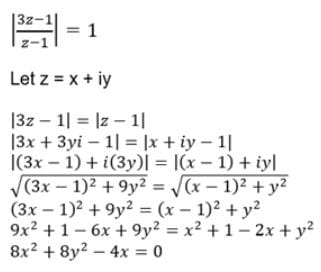This is Equation of a Circle.

Complex Numbers & Quadratic Equations- 1 - Question 25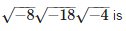Detailed Solution for Complex Numbers & Quadratic Equations- 1 - Question 25

√-8 * √-18 * √-4
= 2√2(√-1) × 3√2(√-1) × 2(√-1)
= 2√2(i) × 3√2(i) × 2(i)
= (2√2 * 3√2 * 2) *(i * i * i)
= (24)(-i2 * i)
= 24(-i)
= -24i

## Mathematics (Maths) Class 11

157 videos|210 docs|132 tests(Scan QR code)